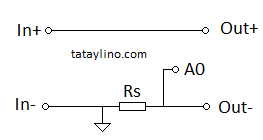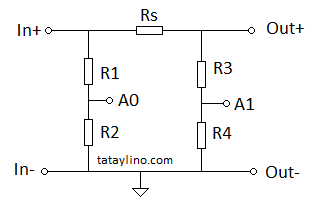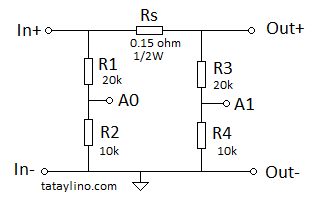# Simple Current Sensor for ArduinoThere is actually a board that you can attach to arduino for measuring current but if you don’t need a very accurate measurement, you can use this very simple circuit. Usually a low resistance resistor is connected in series to the load. The voltage of the resistor is measured and divided to its resistance, the result will be current.

Simple Current SensorSimple approach is just using a single resistor in series to the load, measure the voltage drop of the resistor and divide it by the resistance to get the current. Rs value will depend on the current and voltage drop you need. Take note that the higher the voltage drop the higher the power lost. On the contrary the lower the voltage drop the lower the measurement resolution. So you need to balance on the two.

Compute for value of Rs:

Rs = Vmax/Imax

Where:
Vmax – maximum voltage drop of resistor
Imax – Maximum input current

Compute for Wattage of the Resistor Rs:

Prs = Vmax * Imax or Imax^2 * R

Note: For reliability, it is a good practice to use at least twice resistor wattage(power) than the computed value. For example, if you have computed 0.5W then use at least 1W. This will guaranty that the resistor will not burn.

Compute For Resolution:

Where:
Vref – ADC reference voltage(in arduino it is 5V by default)

Example:
A line with a maximum current of 10A is needing a simple measuring circuit. A resolution of at least 0.1A steps is needed, Compute for the minimum Rs needed.

Resolution is given so we can compute for the Rs:

Re-arrange the equation we get:

Rs = 5/(1023*0.1)

Rs = 0.0488

Since the computed resistor is very small, we will just use a 0.1 ohm that is easier to find.

Compute again for resolution for the new resistor value:

Resolution = 5/(1023*0.1)
Resolution = 0.048A/bit

Compute for Rs power

Prs = Imax^2 * R

Prs   = 10^2 * 0.1

Prs = 10W

Note: Higher resistance will also have higher Power lost. In case you are able to find a 0.051 ohm resistor. The power will be reduced by half(5.1W) but the resolution will be lower 0.095A/bit.

Example Program:

int iin_raw;
double iin_val;

iin_raw = analogRead(0); // 0 = Connected to A0
iin_val = iin_raw * 0.048 // Computed resolution = 0.048A/bit

Positive Side Current Sensor

The solution presented above has problem, you can’t use it to measure charging current simply because the voltage on the Rs reverses when charging and not charging depending where your ground is connected. This won’t be a problem if you have separate supply for your arduino other than the battery being charged. One solution is to put the Rs to the positive side, the drawback though is you will need 2 ADC channels to measure the current. This is not so much an issue because you are also measuring the battery voltage on the other side. One advantage of this circuit is that you can measure the current in either direction so you can have a negative reading.

The resistor dividers(R1-R4) can be omitted if the input voltage is not exceeding 5V. If the input is more than 5V, set the resistor divider values so that the output voltage will be 5V at expected maximum input voltage. Rs should be as low as possible to minimize power loss on the resistor.Note:
* A0 and A1 Corresponds to the analog pin of the arduino.
* Vo is the output of the voltage divider named A0 and A1.
* Vi is the maximum input voltage
* Vo is the Vref of ADC(in arduino it is 5V by default.
* R1 = R3 and R2 = R4

Set R2 and R4 at least 10k to minimize effect on the current consumption. Set R1 and R3 so that the output voltage is 5V when input voltage is at maximum.

Resolution computation is almost the same, except that you will use the max input voltage instead of the Vref:

Example:

Design a simple current measuring circuit to measure charge current of a 12V lead acid battery. Maximum charge current of 1A and a resolution of 0.1A.Set R2 and R4 to 10k

Since 12V lead acid battery voltage can reach up to 14.4V, lets assume the maximum voltage to be 15V.

Compute for R1:

Compute for R1 and R3:

R1 = R2(Vi/Vref – 1)

R1 = 10k(15V/5V – 1)

R1 = 20k (same as R3)

Compute for Rs

Using the equation of the resolution, we can compute for the Rs:

Re-arrange the equation to get Rs:

Rs = 15/(1023*0.1)

Rs = 0.146 ohm

We can use a standard value of 0.15 ohm, compute again for the actual resolution will be:

Resolution = 15/(1023* 0.15)

Resolution = 0.09775 A/bit

Compute for wattage of resistor Rs:

Prs = I^2 * R

Prs = 1^2 * 0.15

Prs = 0.15W

Complete Design:As what I have said, you can use at least twice the computed power so the nearest standard wattage of resistor you can use is 1/2W. But you can also use 1/4W since it is still near the 0.3W requirement. Just be sure that the resistor is well ventilated.

Sample Arduino Program

int iin_raw1;
int iin_raw2;
double iin_val;

iin_raw1 = analogRead(0); // 0 = Connected to A0
iin_raw2 = analogRead(1); // 0 = Connected to A1
iin_val = (iin_raw1 – iin_raw2)  * 0.09775 // Computed resolution = 0.09775A/bit

Final Words
There are current sensor that you can use out there but if you don’t need a very accurate measurement and cost is important then you can use this approach. Balance also the resolution versus power lost on the resistor. Higher resolution requires higher resistances which translate higher energy lost. A solution for the higher resolution for less power lost is using an op-amp to amplify the voltage on the sense resistor Rs, this will be covered on a separate article soon.